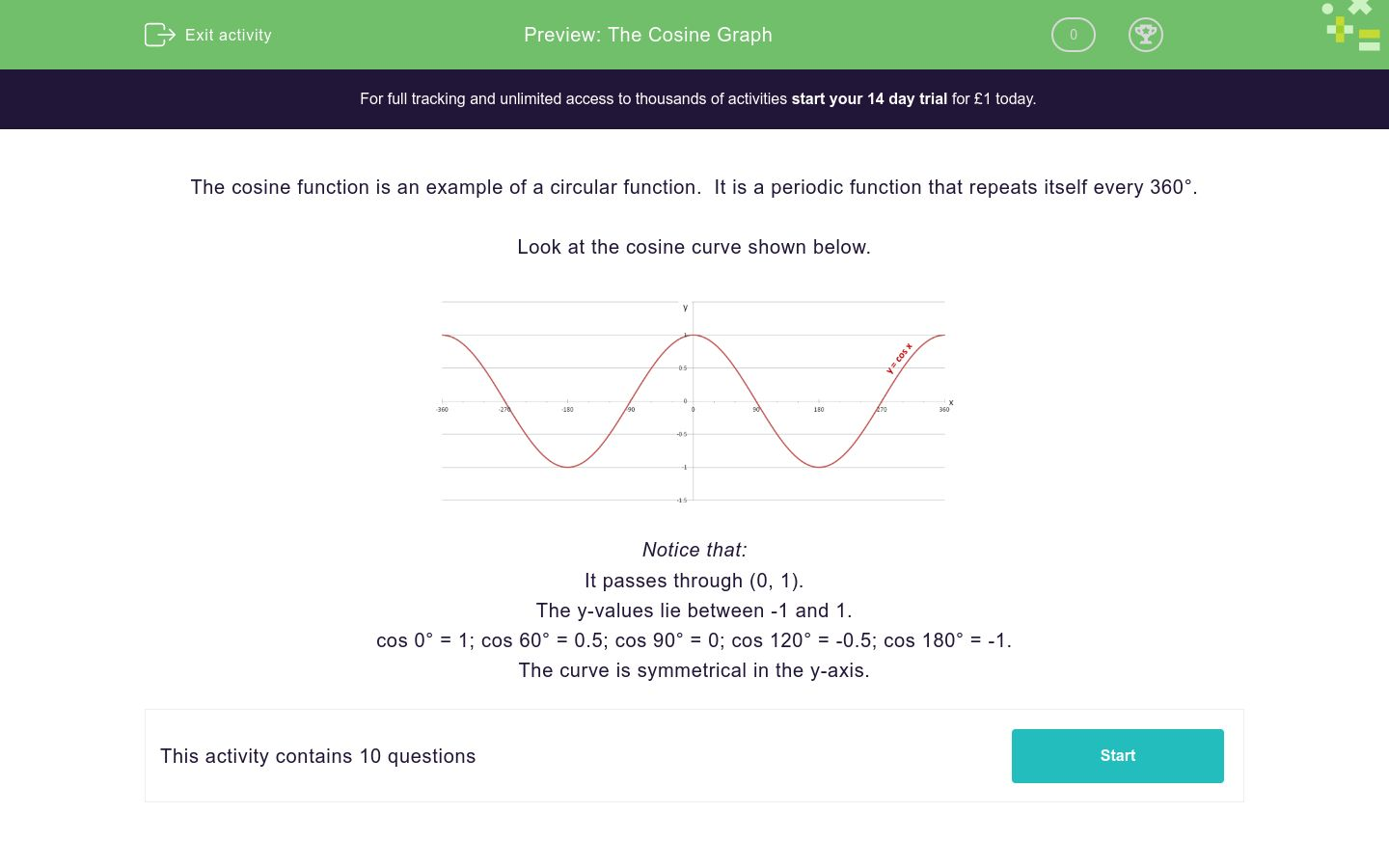# The Cosine Graph

In this worksheet, students read values from a simple cosine graph.Key stage:  KS 4

Curriculum topic:  Geometry and Measures

Curriculum subtopic:  Know the Exact Values of Sin, Cos and Tan

Difficulty level:### QUESTION 1 of 10

The cosine function is an example of a circular function.  It is a periodic function that repeats itself every 360°.

Look at the cosine curve shown below.Notice that:

It passes through (0, 1).

The y-values lie between -1 and 1.

cos 0° = 1; cos 60° = 0.5; cos 90° = 0; cos 120° = -0.5; cos 180° = -1.

The curve is symmetrical in the y-axis.

Use the cosine graph shown below to state the value of:

cos 180°Use the cosine graph shown below to state the value of:

cos 90°Use the cosine graph shown below to state the value of:

cos 270°Use the cosine graph shown below to state the value of:

cos -270°Use the cosine graph shown below to state the value of:

cos -60°Use the cosine graph shown below to state the value of:

cos 240°Use the cosine graph shown below to state the value of:

cos 300°Use the cosine graph shown below to state the value of:

cos -240°Use the cosine graph shown below to state all the angles x for which:

cos x° = 1360

90

30

0

-210

-270

-330

-360

Use the cosine graph shown below to state all the angles x for which:

cos x° = 0.5300

90

60

30

-60

-270

-300

-330

• Question 1

Use the cosine graph shown below to state the value of:

cos 180°-1
EDDIE SAYS
cos 180° = -1
• Question 2

Use the cosine graph shown below to state the value of:

cos 90°0
EDDIE SAYS
cos 90° = 0
• Question 3

Use the cosine graph shown below to state the value of:

cos 270°0
EDDIE SAYS
cos 270° = 0
• Question 4

Use the cosine graph shown below to state the value of:

cos -270°0
EDDIE SAYS
cos -270° = 0
• Question 5

Use the cosine graph shown below to state the value of:

cos -60°0.5
EDDIE SAYS
cos -60° = 0.5
• Question 6

Use the cosine graph shown below to state the value of:

cos 240°-0.5
EDDIE SAYS
cos 240° = -0.5
• Question 7

Use the cosine graph shown below to state the value of:

cos 300°0.5
EDDIE SAYS
cos 300° = 0.5
• Question 8

Use the cosine graph shown below to state the value of:

cos -240°-0.5
EDDIE SAYS
cos -240° = -0.5
• Question 9

Use the cosine graph shown below to state all the angles x for which:

cos x° = 1360
0
-360
EDDIE SAYS
cos -360° = cos 0° = cos 360° = 1
• Question 10

Use the cosine graph shown below to state all the angles x for which:

cos x° = 0.5300
60
-60
-300
EDDIE SAYS
cos -300° = cos -60° = cos 60° = cos 300° = 0.5
---- OR ----

Sign up for a £1 trial so you can track and measure your child's progress on this activity.

### What is EdPlace?

We're your National Curriculum aligned online education content provider helping each child succeed in English, maths and science from year 1 to GCSE. With an EdPlace account you’ll be able to track and measure progress, helping each child achieve their best. We build confidence and attainment by personalising each child’s learning at a level that suits them.

Start your £1 trial